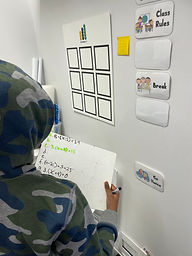## Ms. Melissa

### Target 1​

###### Lesson Type:

Continuation

Algebra

:

Variable

Solve mathematical problems by creating algebraic expressions.

###### 1:

Examine real-world problems and translate those patterns into algebraic expressions.

###### 2:

Use variables to represent numbers and write expression when solving real-world mathematical problems.

###### 3:

Use variable expressions to represent quantities that change in relationship to one another.

6th

###### Vocabulary:

Verbal Expression, Algebraic Expression

Activities:

• Students were given verbal expressions and created algebraic expressions.
• Students were given algebraic expressions and created verbal expressions out of them.
• Students correlated specific words with different operations to support their verbal and algebraic expressions.### Home Exploration

###### Guiding Questions:## Absent Students:

### Target 2

:

###### 1:

Represent inequalities on a number line.

7th

###### Vocabulary:

Inequality, Greater Than or Equal To, Less Than or Equal To

Activities:

• Students discussed the ifference between greater than, less than, greater than or equal to, and less than or equal to.
• Students discussed the correlation with an open or closed circle and inequalities.
• Students worked as a class to create a photo on the computer with the proper characteristics of an inequality.### Home Exploration

###### Guiding Questions:### Target 3

:

###### Vocabulary:

Activities:### Home Exploration